# Online Mechanical Engineering Test - Engineering Mechanics TestLoading Test...

Instruction:

• This is a FREE online test. DO NOT pay money to anyone to attend this test.
• Total number of questions : 20.
• Time alloted : 30 minutes.
• Each question carry 1 mark, no negative marks.
• DO NOT refresh the page.
• All the best :-).

1.

Two balls of equal mass and of perfectly elastic material are lying on the floor. One of the ball with velocity v is made to struck the second ball. Both the balls after impact will move with a velocity

A.
 vB.
 v/2C.
 v/4D.
 v/82.

Coefficient of friction is the ratio of the limiting friction to the normal reaction between the two bodies.

A.
 YesB.
 No3.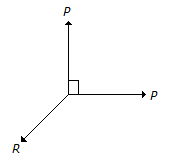The above figure shows the two equal forces at right angles acting at a point. The value of force R acting along their bisector and in opposite direction is

A.
 P/2B.
 2PC.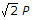D.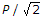4.

The algebraic sum of the resolved parts of a number of forces in a given direction is equal to the resolved part of their resultant in the same direction. This is known as

A.
 principle of independence of forcesB.
 principle of resolution of forcesC.
 principle of transmissibility of forcesD.
 none of these5.

The centre of gravity of an is osceles triangle with base (p) and sides (q) from its base is

A.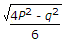B.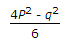C.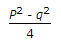D.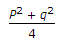6.

The loss of kinetic energy during inelastic impact, is given by(where m1 = Mass of the first body,m2 = Mass of the second body, and u1 and u2 = Velocities of the first and second bodies respectively.)

A.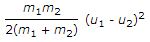B.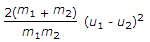C.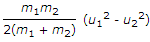D.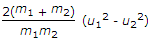7.

The centre of gravity of a hemisphere lies at a distance of 3r / 8 from its base measured along the vertical radius.

A.
 CorrectB.
 Incorrect8.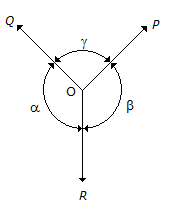The above figure shows the three coplaner forces P, Q and R acting at a point O. If these forces are in equilibrium, then

A.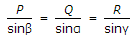B.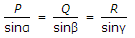C.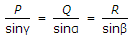D.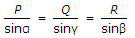9.

The centre of gravity of a right circular solid cone is at a distance of __________ from its base, measured along the vertical axis.(where h = Height of a right circular solid cone.)

A.
 h/2B.
 h/3C.
 h/4D.
 h/610.

The efficiency of a screw jack is maximum, when(where α = Helix angle, and φ = Angle of friction.)

A.
 α = 45° + φ/2B.
 α = 45° - φ/2C.
 α = 90° + φD.
 α = 90° - φ11.

When a train is rounding a curve, the side thrust on the wheel flanges is prevented by raising the outer edge of the rail.

A.
 YesB.
 No12.

The moment of inertia of a thin spherical shell of mass m and radius r, about its diameter is

A.
 mr2/3B.
 2mr2/3C.
 2mr2/5D.
 3mr2/513.

The velocity ratio for the second system of pulleys is n.

A.
 TrueB.
 False14.

The forces which do not meet at one point and their lines of action do not lie on the same plane are known as

A.
 coplaner concurrent forcesB.
 coplaner non-concurrent forcesC.
 non-coplaner concurrent forcesD.
 none of these15.

A body is said to move or vibrate with simple harmonic motion if its acceleration is directed towards the mean position.

A.
 TrueB.
 False16.

A ladder is resting on a smooth ground and leaning against a rough vertical wall. The force of friction will act

A.
 towards the wall at its upper andB.
 away from the wall at its upper endC.
 downward at its upper endD.
 upward at its upper end17.

Two blocks A and B of masses 150 kg and 50 kg respectively are connected by means of a string as shown in the below figure. The tension in all the three strings __________ be same.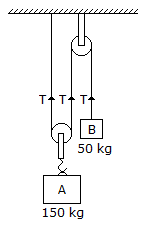A.
 willB.
 will not18.

The forces which meet at one point, but their lines of action __________ on the same plane, are Known as non-coplaner concurrent forces.

A.
 lieB.
 do not lie19.

The force applied on a body of mass 100 kg to produce an acceleration of 5 m/s2, is

A.
 20NB.
 100NC.
 500ND.
 none of these20.

Two equal and opposite parallel forces whose lines of action are different, can be replaced by a single force parallel to the given forces.

A.
 CorrectB.
 Incorrect